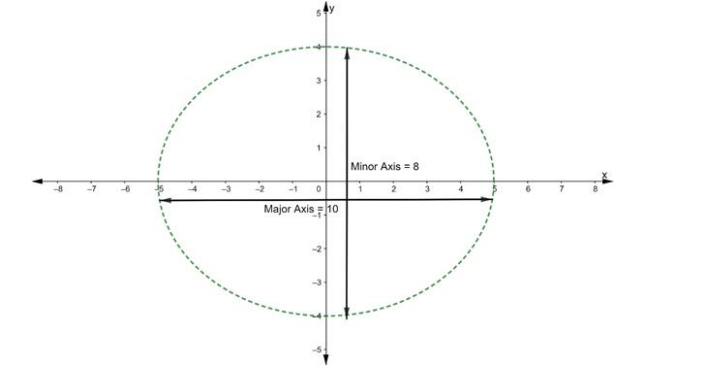# Find the equation of an ellipse, the lengths of whose major and mirror axes`
Question:

Find the equation of an ellipse, the lengths of whose major and mirror axes are 10 and 8 units respectively.

Solution:Let the equation of required ellipse is

$\frac{x^{2}}{a^{2}}+\frac{y^{2}}{b^{2}}=1$ …(A)

Given:

Length of Major Axis = 10units ...(i)

We know that,

Length of major axis $=2 \mathrm{a} \ldots$ (ii)

$\therefore$ From eq. (i) and (ii), we get

2a = 10

⇒ a = 5

It is also given that

Length of Minor Axis $=8$ units ..(iii)

We know that,

Length of minor axis $=2 \mathrm{~b} \ldots$ (iv)

$\therefore$ From eq. (iii) and (iv), we get

2b = 8

⇒ b = 4

Substituting the value of a and b in eq. (A), we get

$\frac{x^{2}}{(5)^{2}}+\frac{y^{2}}{(4)^{2}}=1$

$\Rightarrow \frac{x^{2}}{25}+\frac{y^{2}}{16}=1$